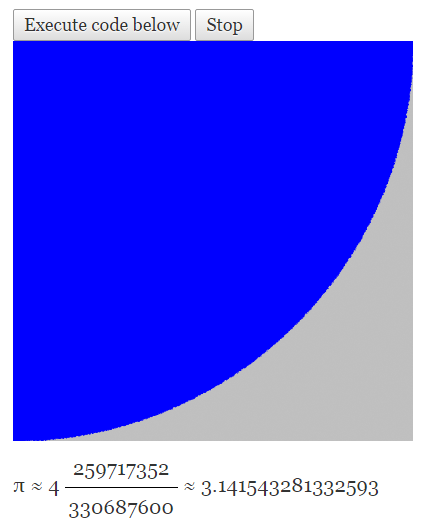{{announcement.body}}
{{announcement.title}}

# The Randomness of Being: Part 1

DZone 's Guide to

# The Randomness of Being: Part 1

### Knowing what to expect is a good most of the time ... except when we are trying to generate random numbers. Nearly all computer languages have a random number function, but is it random enough? What is random? What should we be concerned about?

· Web Dev Zone ·
Free Resource

Comment (0)

Save
{{ articles.views | formatCount}} Views

Random numbers are used all the time in computer science. My background is mostly scientific and engineering where random numbers are used quite often for Monte Carlo simulation techniques. The domain data space is too vast to exhaustively test all the possibilities so in order to explore solutions the simulation randomly distributes the values of the input parameters generating a large number of output results which can be validated with various statistical tests. For instance you can calculate the value of pi using random numbers in this way.

[If you weren't aware it could be done like this you owe it to yourself to give it a few minutes of thought. There are lots of interesting methods on the Internet. Some of the more interesting ones involve throwing darts! Here is a good example of how to do it in your browser and it even includes an example with graphic JavaScript. ]

Once you figure it out (or take the easy way out and read about it) the method seems elegant and sound. But it does have a potential flaw. It relies heavily on the true randomness of the numbers used to generate the Monte Carlo test points. The algorithm expects the distribution of the probability of the random number generator to be flat. If you are generating random floating-point numbers between 0.0 and 1.0, then the odds of any number appearing must be exactly the same as any other number. If for example numbers around 0.5 were even slightly more likely to occur than other numbers, then your final solution will be biased. It will converge on an incorrect answer. In the JavaScript example I referenced above it's easy to see that more points would be placed within the circular arc, and since the point count represents the area of the circle it follows that the ratio of the areas of the circle and the square would be too big. Therefore, the value computed for pi would be too big also.

[Another issue to consider when thinking about flatness of the distribution is: How big of a sample do you take. If it's flat over the sample then was it flat over the first half of the sample and the second half of the sample? If you do run the JavaScript example you may find it interesting to watch how the estimated value of pi wanders over minutes or hours. Maybe the JavaScript random number generator isn't perfect?]

So, it is critical that random numbers not only be jumbled and without any apparent order, but they must be equally likely to occur (flat distribution) in order for any Monte Carlo technique to work properly.

Most random number generators provided as a part of whatever computer language you're using is not really random. They are software algorithms and as such they are deterministic. These random functions we use are usually referred to as pseudorandom number generators. And they have been engineered to do a reasonably good job producing a flat distribution of jumbled numbers.

But is that enough? I will address that in Part 2

FYI: After 12 hours of JavaScript Monte Carlo simulation the value of pi seems to be 3.14154xxxxxxx.Is this bias acceptable? Will the answer get better with another 12 hours? These are random questions for you to consider.

Topics:
random numbers ,cryptography ,monte carlo ,statistical analysis

Comment (0)

Save
{{ articles.views | formatCount}} Views

Opinions expressed by DZone contributors are their own.